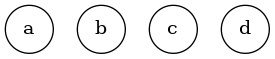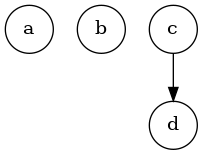# Play With Lua!

## Enumerating All Trees

This is a problem in The Art of Computer Programming volume 4A, which only came out a few months ago. My solution isn’t Knuth’s solution, mostly because I don’t understand Knuth’s solution, but it works and it’s fairly easy to explain.

The problem is this: given a number of nodes (like, say, 4), generate every possible arrangement of those nodes into a tree. So this is one:

`() () () ()`This is another one:

`() () (())`And so on. For n nodes there are 2n-1 possible trees. And it’s not immediately obvious how to generate them.

Knuth’s solution builds the strings directly, through some magic I don’t quite understand. I think he’s doing the same general thing I am, but he’s got a way to do it directly, whereas I’m going to build the trees in memory first, out of Lua tables, and then print strings from them.

So, first I want a simple way to print the strings. This function takes a table and returns the parentheses that represent it:

```function tree_str(tree)
if #tree == 0 then return ""
else
local subtrees = {}

for _, subtree in ipairs(tree) do
table.insert(subtrees, "(" .. tree_str(subtree) .. ")")
end

return table.concat(subtrees, " ")
end
end```

We can test it like this:

`tree_str({ {}, {}, {{}, {}}}) --&gt; "() () (() ())"`

Simple enough. Now that that’s out of the way, how do we actually do this? Well, let’s first try to figure it out inductively. If we have the set of trees for n-1 nodes, and we add an nth node, we can figure it will double the number of trees: each tree of n-1 can either be a descendent of the new node or a sibling of it.

Since there is only one tree for the first node, and each one doubles the count, there are 2n-1 trees. If we can figure out some 1-to-1 correlation between bit fields and trees, we can just count from 1 to 2n-1-1 and generate the tree for each one. So, how to do that?

The way I figured out is, each node’s bit determines whether it’s a sibling or a child of the previous node. We keep a `current_node` reference, and if the bit is zero, we push a new table into it; if it’s 1, we find `current_node`‘s last child, make that the new `current_node`, and then add a child to that.

Right away we run into a problem, because Lua doesn’t have any bitwise operators. So let’s make a quick function to dump out all the bits in a number:

```function bits(n)
local b = {}
while n &gt; 0 do
b[#b+1] = n%2
n = (n - n%2) / 2
end
return b
end```

We don’t care what the indices are, so we may as well have them be 1-indexed so that we can use `ipairs`. We can use this function to generate all the trees, like this:

```function make_tree(node_count, n)
local b = bits(n)
local nodes = { {} }
local current_node = nodes

while node_count &gt; 1 do
node_count = node_count - 1
if b[node_count] == 1 then
current_node = current_node[#current_node]
end
current_node[#current_node + 1] = {}
end

return nodes
end```

The loop is structured a little weirdly because we want to skip the first node; it can’t possibly be a child of the previous node even if it’s a 1.

So now, we can generate a single tree, so let’s loop through to make all the trees:

```for n = 0,7 do
local str = tree_str(make_tree(4, n))
print(str)
end```

And we see:

```() () () ()
() () (())
() (() ())
() ((()))
(() () ())
(() (()))
((() ()))
(((())))```

And there, we’ve enumerated all the possible trees with four nodes! Here’s the code.

Written by randrews

May 17th, 2011 at 10:24 pm

Posted in Uncategorized